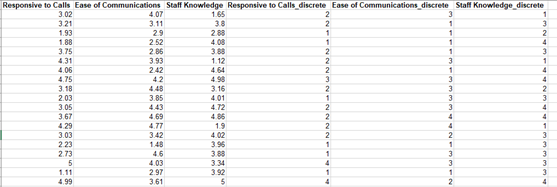Include Top

# Convert to Discrete

This tool is used to convert continuous data to discrete (a.k.a. discretization) using percentile based equal frequency. It is useful for graphical and statistical tools that require group category variables.

The percentiles are selected to produce the specified number of discrete levels. For example, with the default number of discrete levels = 4, SigmaXL uses the 25th percentile, 50th percentile and 75th percentile as bin intervals. The numerical values are converted as follows:

Minimum to < 25th percentile -> 1
25th percentile to < 50th percentile -> 2
50th percentile to < 75th percentile -> 3
75th percentile to Maximum -> 4

These percentiles will produce an approximate equal frequency if the data are continuous. Ties will result in some unequal frequency counts.

1. Click Sheet 1 tab of Customer Data.xlsx.
2. Click SigmaXL > Data Manipulation > Convert to Discrete. Ensure that the entire data table is selected. If not, check Use Entire Data Table. Click Next.
3. Select Responsive to Calls, Ease of Communications and Staff Knowledge. Click Numeric Data Variables (Y) >>.4. Click OK. The results are given on the Convert to Discrete sheet:# Web Demos

Our CTO and Co-Founder, John Noguera, regularly hosts free Web Demos featuring SigmaXL and DiscoverSim